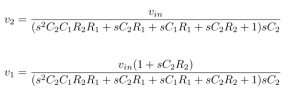### PNP Transistor as a Current Source

• We wanted to use a transistor as a current source.
• Two resistors, one at the emiiter(R1) and the other at the collector(R2) were connected.
• A voltage divider circuit with a supply of 9v was connected at the base that could supply certain voltage.

Constraints :

• We wanted 4.5mA of current to pass through.
• 9v supply was given to the resistor at the emitter.

Design :

• The resistor at the collector(R2) was chosen as 1k based upon the resistance of the mic.
• We knew that the diode would turn on at around 0.7v.
• The voltage drop across R1 should be some resistance that gives 4.5mA of current.
• Depending upon the availability of the resistors, 330 ohms was chosen for R1, keeping the 9v supply in mind and voltage at emiiter, it was calculated that 1.5v drop would give us 4.5mA of current.
• Now from the voltage divider, a certain amount of voltage needed to be supplied so that the drop across R1 is 1.5v (ie, 9v – 7.5v)
• So we wanted 6.8v from the voltage divider (ie, 6.8v + 0.7v = 7.5v)
• Hence, the voltage divider circuit was designed with the following formula.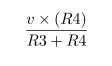• The ratio of the scaling factor was approximately 3 and depending upon the availability of the resistors, R3 was taken as 4.7k and R4 was taken as 14.5k.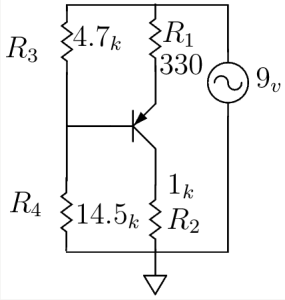### Amplifier – Simulation and Results

First started with constructing constant current source using PNP transistor with 9V supply voltage.

voltage reading:    3.32V                                               current reading:332mA

AMPLIFIER:
Added one more transistor on NPN in the circuit with the same supply voltage of 9V and input signal of 0.2V.

Simulation Result: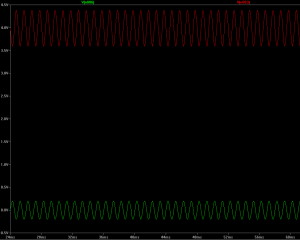Red –> Amplified Output Voltage

Green –> Input Voltage

Schematic diagram:

In order to increase the gain of the Amplifier, added one capacitor C1 parallel with R6 resistor.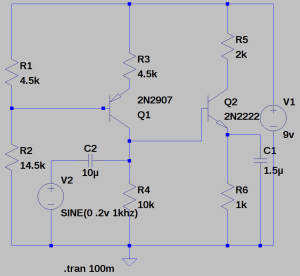Red –> Amplified Output Voltage

Green –> Input Voltage

### SpeeDe V_03

SpeeDe V_03:
The aim of the third version of the SpeeDe is to improve the sensitivity to capture the speed of even smaller object. In this regard, I have done some modification in the program ( using the concept of interrupts in Arduino).

ARDUINO PROGRAM:                                                                                                                       // include the library code:
#include <LiquidCrystal.h>
// constants won’t change. They’re used here to
// set pin numbers:
// variables will change:
volatile int State = 0;         // variable for reading the pushbutton status
// Start and stop times
volatile unsigned long start_time_us = 0;
volatile unsigned long stop_time_us = 0;
float delta_time;
float speed_var;

See More

Reference:                                                                                                                             http://www.auraauro.com/uncategorized/speede-v-01-2/
http://arduino.cc/en/Reference/attachInterrupt

### Sampling Theorem

SAMPLING:   A continuous time signal can be represented in its samples and can be recovered back when sampling frequency fs is greater than or equal to the twice the highest frequency (fm) component of message signal. i. e  fs 2fm.

Consider a continuous time signal x(t). The spectrum of x(t) is a band limited to fm Hz. Sampling of input signal x(t) can be obtained by multiplying x(t) with an impulse train δ(t) of period Ts. The output of multiplier is a discrete signal called sampled signal which is represented with y(t) in the following diagrams: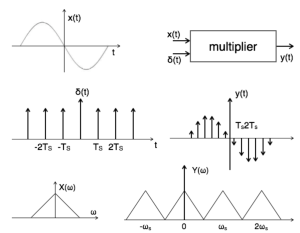ALIASING:

Different signals to become indistinguishable (or aliases of one another) when sampled is called Aliasing. Aliasing occurs when a system is measured at an insufficient sampling rate.Reference:

http://www.tutorialspoint.com/signals_and_systems/signals_sampling_theorem.htm

### Demonstration of Division

Many  teachers struggle with teaching division to children and I have been thinking about this recently. I think there are a number of factors contributing to this. Firstly,  there is a huge range of level of understanding of the concept of division and secondly the concept itself can be thought of as building on children’s understandings of addition, subtraction and multiplication. The spread of different levels of understanding is likely to make any class “mixed ability” for this topic. There are also difficulties with the written algorithm. Altogether, it is a complicated picture. So I Started to create the division program in Scratch to give demonstration.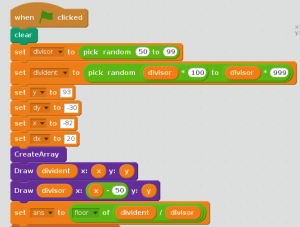See more : https://scratch.mit.edu/projects/56911106/

### At Isai Ambalam

For the first few weeks I decided to teach and learn with the 5th graders at Isai Ambalam middle school, at class we worked out many logic puzzles. The class was at the stage of working out multiplication and division. Speed,distance,time and interrelating each other to form stories of multiplication and division were introduced…

e.g.: if a car travels at a speed of 25 km/hr, what will be the distance covered by the car in 4 hours?

The students multiplied and came up with a solution, by saying that the car would cover a distance of 100kms. The same story was made into division

e.g.: if a car covers 100km in 4hours, what is the speed of the car? The speed of the car is 25km/hr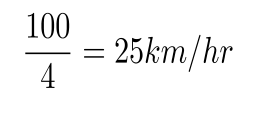the students were able to make out the stories, as we got into creating many different stories I started to realize that not all students did understand what they were making up, some got confused or did not understand the units..

I asked the students how far was their home from school, well there were many loud answers, but each answer terminated with a unit of distance( the lesson learn ‘t was to never ask a general questions to the whole class, or else expect a chorus answer if you don’t want that to happen, be more specific and select a random child to question) . They all knew that time was in hours, but the units of speed and distance always seemed to confuse them until they came to realize that speed is the amount of distance covered over a certain period of time….

the real reason was that the majority of the students did not understand English very well, being bilingual is very very important. But once the conversation starts in Tamil( mother tongue) its easy to forget the conscious of switching back to English…

by the time these few weeks passed the relation with the class grew stronger, I came to realize that there were some four students who seriously did not know what they were doing in mathematics class… every class has a few slow learners, but having students who could not multiply, or even added by using their fingers seemed a little bit odd. I decided to take into consideration these four members of the classroom.

The five of us would sit together and start to solve some multiplication problems, after a while they seemed to get a handle of the multiplication… but the problem showed up in the addition part after multiplying two, two digit numbers… simple additions required finger counting, they could not do mental calculations. If asked what is five plus six they would take five fingers and then start to count six fingers, to see that number 1 was left after adding five plus five and the answer was 11 was not that obvious.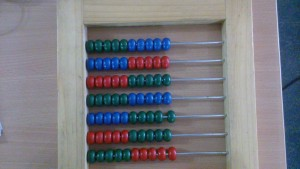To create a change the abacus was introduced and still it was a little hard to see the fives, as the whole ten beads were the same colour. Now the abacus was taken apart and every five beads were altered so as to see the simple pattern of fives. After this, the pattern was seen that when one adds five to seven the remaining number of beads is two and the answer is twelve. To have broken the pattern of finger calculation which they were stuck with for all these years felt awesomely superb. But after a few days with the abacus rigorous training was difficult and the student seemed to be getting a littler tired of beads counting….

that is when introducing scratch programming seemed to ignite a little spark, as a team we came together and built a small script that added two digit numbers. The children were so excited and eager to solve the sums in scratch than before with the abacus. Now doing mental calculations seemed to easier than before.

https://scratch.mit.edu/projects/56560028/

### Scratch stories with Udavi 8th graders

Scratch stories with Udavi 8th graders

The 8th graders at Udavi had made stories on the theme ‘ if i had wings ‘ , they were wanting to depict their stories with scratch. The time offered was four English class hours on that week, and so the fun began… on the first day all the students had worked through an idea of what they wanted to depict with scratch programming, and when asked to pair up as a team and do their work, the usual boy-boy and girl -girl teams appeared. To make things more collaborative and interesting we mixed up the pairs into boy – girl.., asked on what aspects , criteria they were willing to do evaluate the work. They came up with the following

-Colourful

-Creative

-Beautiful

-Using Proper Language

-Interesting

-Teamwork

The class was just so amazing, all the children were totally focused on their work as a team there were no cross talks between teams.The students used the Internet to get their characters and backgrounds. Gimp was introduced to them as and editing tool.On the 2nd and 3rd day the children continued working with their script. They were also engaged in giving feedback with the mentioned aspects. Towards the end of the last assigned day all the projects were decided to be merged as to make a single video. The students amongst themselves coordinated and went along other teams that were still engaged in their scripting, and started to explain the process of merging files.

### Incremental Backup for Ubuntu

The command :

tar -cvfz -X excludeList -g 20150407.snar 20150407-full.tar.gz ~

tar-cvfz – creates all the files.

-X excludeList – creates a folder where one can exclude the files that need not be backed up.

-g .snar – this is the command used for incremental backup.

.tar.gz ~ – create .gz files which can be extracted later

• At first do a full blind backup of all your files.
• Then for the next backup, create a new .snar file and copy the old .snar to the new one.

### Calculating ESL of a Capacitor

Impedance of Various 100μF Capacitors :• The figure tells us that the impedance of a capacitor will decrease monotonically as frequency is increased.

• In actual practice, the ESR causes the impedance plot to flatten out.

• As we continue up in frequency, the impedance will start to rise due to the ESL of the capacitor.

• The location and width of the “knee” will vary with capacitor construction, dielectric and value.

• This is why we often see larger value capacitors paralleled with smaller values. The smaller value capacitor will typically have lower ESL and continue to “look” like a capacitor higher in frequency.

• This extends the overall performance of the parallel combination over a wider frequency range.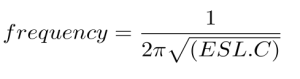Reference : From Analog Devices Tutorial

Frequency Characteristics of a 0.1 uf Capacitor :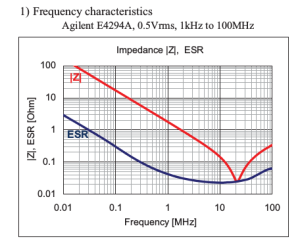The impedance matches with ESR at around at around 2 Mhz.

ESL Analysis :

Frequency = 20 Mhz

Capacitance = 0.1 uF

From the frequency equation , ESL = 0.63 nH

Frequency Characteristics of a 1 uf Capacitor :

The impedance matches with ESR at around at around 8 Mhz.

ESL Analysis :

The impedance matches with ESR at around at around 8 Mhz.

Frequency = 8 Mhz

Capacitance = 1 uF

From the frequency equation , ESL = 0.39 nH

The impedance matches with ESR at around at around 2 Mhz.

ESL Analysis :

Frequency = 2 Mhz

Capacitance = 10 uF

From the frequency equation , ESL = 0.63 nH

Frequency Characteristics of a 10uf Capacitor :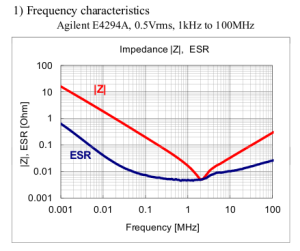The impedance matches with ESR at around at around 2 Mhz.

ESL Analysis :

Frequency = 2 Mhz

Capacitance = 10 uF

From the frequency equation , ESL = 0.63 nH

Capacitor Graph Reference : Datasheets from Digikey

### CIRCUIT ANALYSIS

CIRCUIT ANALYSIS:
Lets analysis the following circuit.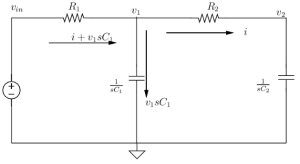This circuit consist of two nodes named as v1 & v2
Consider the input voltage is vin .
Lets say the current ‘i’ is passing through the node v2 .
Then the voltage at the node v2 is given by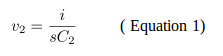Voltage at the node V1 is given by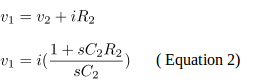Then the input voltage vin is given by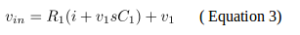Substituting the value of V1 in equation 3 we get,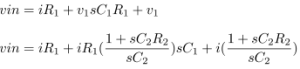(Equation 4)

Then the current ‘i’ passing through the node V1 is,(Equation 5)

The transfer function (by substituting the value of ‘i’ in equations 1 & 2)